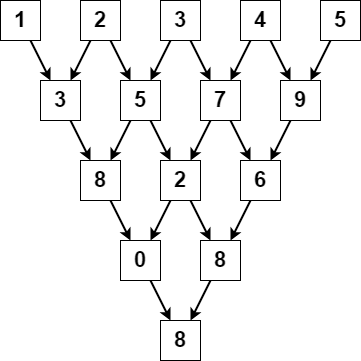2221. Find Triangular Sum of an Array

#### QUESTION:

You are given a 0-indexed integer array nums, where nums[i] is a digit between 0 and 9 (inclusive).

The triangular sum of nums is the value of the only element present in nums after the following process terminates:

Let nums comprise of n elements. If n == 1, end the process. Otherwise, create a new 0-indexed integer array newNums of length n - 1. For each index i, where 0 <= i < n - 1, assign the value of newNums[i] as (nums[i] + nums[i+1]) % 10, where % denotes modulo operator. Replace the array nums with newNums. Repeat the entire process starting from step 1. Return the triangular sum of nums.

Example 1:``````Input: nums = [1,2,3,4,5]
Output: 8
Explanation:
The above diagram depicts the process from which we obtain the triangular sum of the array.
``````

Example 2:

``````Input: nums = 
Output: 5
Explanation:
Since there is only one element in nums, the triangular sum is the value of that element itself.
``````

Constraints:

``````1 <= nums.length <= 1000
0 <= nums[i] <= 9
``````

#### SOLUTION:

``````class Solution {
func triangularSum(_ nums: [Int]) -> Int {
var sums = nums
var length:Int = sums.count
while length != 1 {
for index in 0..<sums.count - 1 {
sums[index] = (sums[index] + sums[index+1]) % 10
}
length -= 1
}
return sums
}
}
``````